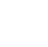Back to Chapter
Getting StartedBasic Syntax & DeclarationControl StatementsNo items found.
Function & EventsNo items found.
Array and its TypesNo items found.
OOP : Object Oriented ProgrammingNo items found.
Javascript StandardsNo items found.
HTML DOMNo items found.No items found.No items found.No items found.

# Array Function

There are Many Predefined Methods / Functions in the Array, that are very helpful to perform certain actions.

For Example: If You want to Filter Out the Elements of Array whose Value is Greater than 50, then how would you do this? To Achieve This We will use some predefined functions. Let's See them in Details.

## Array.some()

The some() method is used to check if any of the Array Element value pass a test Function.

With This Function, we will check is there any Person whose age is greater then 50.

Example:

``` var age = [20, 48, 96, 73, 9]; var result = age.some(myFunction); function myFunction(value) { return value > 50; } console.log(result); // Output // true ```

Here,

It will check for all the elements of the Array. If the Value of Any Element is greater then 50 then it will return True otherwise, it will return False

In this example,

20 will fail

48 will fail

96 will pass because it is greater then 50

So It will Return True.

## Array.find()

The find() method is used to filter the values that passes a specific function.

Now we will find the Solution of Above Question, We will print the First Elements whose age is greater then 50.

Example:

``` var age = [20, 48, 96, 73, 9]; var finalList = age.find(myFunction); function myFunction(value, index, array) { return value > 50; } console.log(finalList); // Output // 96 ```

Here,

It will Print the First Elements which pass the myFunction

i.e. 20 will fail

48 will fail

96 will pass

so it will not check further and print 96

Note : Function here can takes 3 arguments:

• The item value
• The item index
• The array itself

Now, You must be Wondering, what if we want to print all the numbers whose age is above 50 then what will we do? Let's Check It Out!!!

## ForEach()

Javascript Provides forEach Method, by which you can achieve above task.

Example:

``` var age = [20, 48, 96, 73, 9]; age.forEach(myFunction); function myFunction(value) { if(value > 50){ console.log(value); } } // Output // 96 // 73 ```

All the Values that pass through the Function will be Printed.

i.e. 20 will Fail

48 will Fail

96 will Pass

73 will Pass

9 will Fail.

## Array.findIndex()

If you want to Find the Index of First Array Elements that passes a Test Function. To Achieve This you can use findIndex() Method.

We will find the index of 1st Person whose age is above 50.

Example:

``` var age = [20, 48, 96, 73, 9]; var finalList = age.findIndex(myFunction); function myFunction(value, index, array) { return value > 50; } console.log(finalList); // Output // 2 ```

Here,

We will try to understand with the help of Table.

Note : Function here can take 3 arguments:

• The item value
• The item index
• The array itself

## Array.filter()

The filter() method is used to filter the Elements that passes Test Function and Store them to new Array.

In This Example, We will store all the Elements Whose Age is Greater then 50 to the New Array.

Example:

``` var age = [20, 48, 96, 73, 9]; var finalList = age.filter(myFunction); function myFunction(value, index, array) { return value > 50; } console.log(finalList); // Output // [96, 73] ```

Here,

We will try to understand with the help of Table.

Test Cases:

At Last It will Print the finalList Array. You can also use For Loop to Print the finalList Array.

Note : Function here can take 3 arguments:

• The item value
• The item index
• The array itself

## Array.map()

The map() method is used to creates a new array by performing a specific function on each array element, it does not perform any task for the blank elements.

Now, We will find the square of all array elements.

Example:

``` var numbers = [2, 4, 6, 8, 10]; var sqNumbers = numbers.map(myFunction); function myFunction(value, index, array) { return value * value; } console.log(sqNumbers) // Output // [4, 16, 36, 64, 100] ```

Here,

We will try to understand with the help of Table.

Test Cases:

At Last It will Print the sqNumbers Array. You can also use For Loop to Print the sqNumbers Array.

Note : Function here can take 3 arguments:

• The item value
• The item index
• The array itself